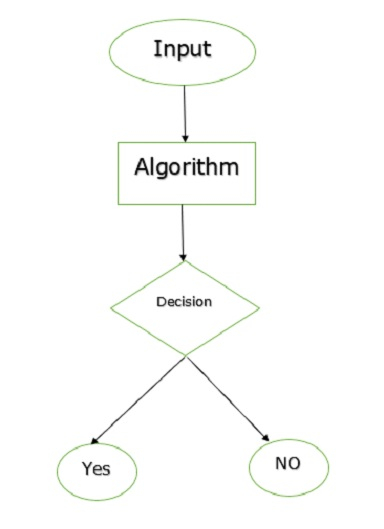# What is the decision problem in TOC?

A decision problem Q is a set of questions, each having either yes or no answer. Let us consider the question is “is 10 a perfect square?” which is an example for a decision problem. A decision problem generally consists of an infinite number of related questions.

## Example

The problem PSQ which determines whether an arbitrary natural number is a perfect square or not having some doubtful questions like the following −

• q0: Is 0 a perfect square?
• q1: Is 1 a perfect square?
• q2: Is 2 a perfect square?

## Solution

A solution to a decision problem Q is an algorithm which determines the approximate answer to every question q ϵ Q.

An algorithm which solves a decision problem has to be the following −

• Complete.
• Mechanistic.
• Deterministic.

A procedure which satisfies the preceding properties is generally called effective.

A problem is called as a decidable if it has a representation in which the set of accepted input strings from a recursive language.

Since computations of deterministic multi track and multi tape machines are simulated on a standard Turing machine, a solution using these machines also establishes the decidability of a problem.

The decision problem will be as follows −